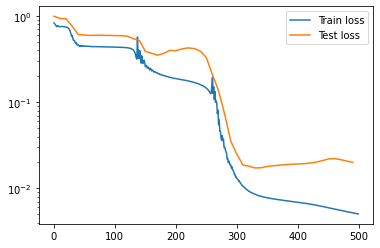# Using EMLP in PyTorch¶

So maybe you haven’t yet realized that Jax is the best way of doing deep learning – that’s ok!

You can use EMLP and the equivariant linear layers in PyTorch. Simply replace import emlp.nn as nn with import emlp.nn.pytorch as nn.

If you’re using a GPU (which we recommend), you will want to set the environment variable so that Jax doesn’t steal all of the GPU memory from PyTorch. Note that if a GPU is visible under CUDA_VISIBLE_DEVICES, you must use the PyTorch EMLP on the GPU.

:

%env XLA_PYTHON_CLIENT_PREALLOCATE=false

env: XLA_PYTHON_CLIENT_PREALLOCATE=false

:

import torch
import emlp.nn.pytorch as nn

:

from emlp.reps import T,V
from emlp.groups import SO13

repin= 4*V # Setup some example data representations
repout = V**0
G = SO13() # The lorentz group

device = torch.device('cuda' if torch.cuda.is_available() else 'cpu')

:

x = torch.randn(5,repin(G).size()).to(device) # generate some random data

model = nn.EMLP(repin,repout,G).to(device) # initialize the model

model(x)

:

tensor([[-0.0039],
[-0.0041],
[-0.0042],
[-0.0040],


The model is a standard pytorch module.

:

model

:

EMLP(
(network): Sequential(
(0): EMLPBlock(
(linear): Linear(in_features=16, out_features=419, bias=True)
(bilinear): BiLinear()
(nonlinearity): GatedNonlinearity()
)
(1): EMLPBlock(
(linear): Linear(in_features=384, out_features=419, bias=True)
(bilinear): BiLinear()
(nonlinearity): GatedNonlinearity()
)
(2): EMLPBlock(
(linear): Linear(in_features=384, out_features=419, bias=True)
(bilinear): BiLinear()
(nonlinearity): GatedNonlinearity()
)
(3): Linear(in_features=384, out_features=1, bias=True)
)
)


## Example Training Loop¶

Ok what about training and autograd and all that? As you can see the training loop is very similar to the objax one in Constructing Equivariant Models.

:

import torch
import emlp.nn.pytorch as nn
from emlp.groups import SO13
import numpy as np
from tqdm.auto import tqdm
from emlp.datasets import ParticleInteraction

trainset = ParticleInteraction(300) # Initialize dataset with 1000 examples
testset = ParticleInteraction(1000)

device = torch.device('cuda' if torch.cuda.is_available() else 'cpu')
BS=500
lr=3e-3
NUM_EPOCHS=500

model = nn.EMLP(trainset.rep_in,trainset.rep_out,group=SO13(),num_layers=3,ch=384).to(device)

def loss(x, y):
yhat = model(x.to(device))
return ((yhat-y.to(device))**2).mean()

def train_op(x, y):
lossval = loss(x,y)
lossval.backward()
optimizer.step()
return lossval

test_losses = []
train_losses = []
for epoch in tqdm(range(NUM_EPOCHS)):
if not epoch%10:


Ok so it’s not nearly as fast as in Jax (maybe 15x slower), but hey you said you wanted PyTorch

:

import matplotlib.pyplot as plt
plt.plot(np.arange(NUM_EPOCHS),train_losses,label='Train loss')
plt.plot(np.arange(0,NUM_EPOCHS,10),test_losses,label='Test loss')
plt.legend()
plt.yscale('log')Bonus: Try out model=nn.MLP(trainset.rep_in,trainset.rep_out,group=SO13()).to(device) and see how well it performs on this problem.

## Converting Jax functions to PyTorch functions (how it works)¶

You can use the underlying equivariant bases $$Q\in \mathbb{R}^{n\times r}$$ and projection operators $$P = QQ^\top$$ in pytorch also.

Since these objects are implicitly defined through LinearOperators, it is not as straightforward as simply calling torch.from_numpy(Q). However, there is a way to use these operators within PyTorch code while preserving any gradients of the operation. We provide the function emlp.reps.pytorch_support.torchify_fn to do this.

[ ]:

import jax
import jax.numpy as jnp
from emlp.reps import V
from emlp.groups import S


For example, let’s setup a representation $$S_4$$ consisting of three vectors and one matrix.

:

W =V(S(4))
rep = 3*W+W**2


First we compute the equivariant basis and equivariant projector linear operators, and then wrap them as functions.

:

Q = (rep>>rep).equivariant_basis()
P = (rep>>rep).equivariant_projector()

:

applyQ = lambda v: Q@v
applyP = lambda v: P@v


We can convert any pure pytorch function into a jax function by applying torchify_fn. Now instead of taking jax objects as inputs and outputing jax objects, these functions take in PyTorch objects and output PyTorch objects.

:

from emlp.nn.pytorch import torchify_fn
applyQ_torch = torchify_fn(applyQ)
applyP_torch = torchify_fn(applyP)


As you would hope, gradients are correctly propagated whether you use the original Jax functions or the torchified pytorch functions.

:

x_torch = torch.arange(Q.shape[-1]).float().cuda()
x_jax  = jnp.asarray(x_torch.cpu().data.numpy())

:

Qx1 = applyQ(x_jax)
Qx2 = applyQ_torch(x_torch)
print("jax output: ",Qx1[:5])
print("torch output: ",Qx2[:5])

jax output:  [0.48484263 0.07053992 0.07053989 0.07053995 1.6988853 ]
torch output:  tensor([0.4848, 0.0705, 0.0705, 0.0705, 1.6989], device='cuda:0',


The outputs match, and note that the torch outputs will be on whichever is the default jax device. Similarly, the gradients of the two objects also match:

:

torch.autograd.grad(Qx2.sum(),x_torch)[:5]

:

tensor([-2.8704,  2.7858, -2.8704,  2.7858, -2.8704], device='cuda:0')

:

jax.grad(lambda x: (Q@x).sum())(x_jax)[:5]

:

DeviceArray([-2.8703732,  2.7858496, -2.8703732,  2.7858496, -2.8703732],            dtype=float32)


So you can safely use these torchified functions within your model, and still compute the gradients correctly.

We use this torchify_fn on the projection operators to convert EMLP to pytorch.